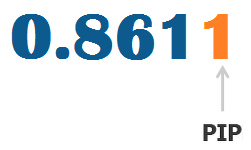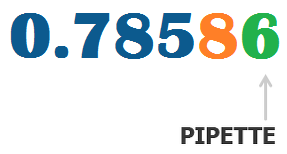• Become a partner
• 0008004430039
• The term Pip is an acronym for “price interest point” or "Percentage in Point." In the Forex market, a pip is the unit of measurement to express the change in value between two currencies.

### What Is a Pip and a Pipette?

For example, seeing the AUD/USD quote going from 0.8611 to 0.8610, we would call that a price decline of 0.0001, or 1 pip. If the current price of USD/JPY is 118.13 and it goes to 118.14, this is a price rise of 0.01, or 1 pip. Thus, one pip is the smallest change in value for a quote that allows two or four decimal places.Recently, more and more brokers have gone beyond the standard four and two decimal places, offering quotes with five and three decimal places. This third or fifth decimal is regarded as Pipette. A Forex broker could quote the NZD/USD pair as 0.78586. If that goes to 0.78587, the movement is termed as a 1 pipette rise in value.### How to Calculate the Value of One Pip

The calculation of the value of one pip varies from one currency to another. The difference comes from examining whether the U.S. dollar is the base currency or the quote currency. The following two examples will illustrate just that.

### U.S. dollar as base currency

The price of USD/JPY is read as 1.1332. Thus, the smallest movement for a pip is 0.0001. The formula to calculate the value of one pip is: Pip Value = Smallest decimal move / Current exchange rate = 0.0001 / 1.1332 = 0.000882

Thus, the value of 1 pip when the USD/JPY is at 1.1332 is USD 0.000882.

### U.S. dollar as quote currency

The price of EUR/USD is 0.8613. The smallest movement for a pip is 0.0001. We will also calculate the value of one pip using the above formula which is:

Pip Value = Smallest decimal move / Current exchange rate = 0.0001 / 0.08613 = 0.001161

Of course, you do not need to work this out yourself, as Forex4you will always do this calculation for you automatically. Since it is integrated into the trading platforms of Forex4you, there is no need to calculate it manually.